Because of noise on the channel, the received waveform will never be exactly, identical to the transmitted waveform. In order to minimize the probability that the receiver detects a bit in error, we use the following metric to determine whether we have received a zero or a one: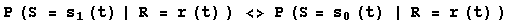If, given the received signal, the probability that the transmitted signal was a one is greater than the probability that the transmitted signal was a zero, we assume the transmitted signal was a one. This probability can be re-expressed as follows: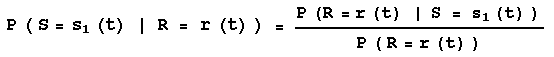Since the denominator is common to both sides of Eqn. #1, it can be ignored. The numerator can be expressed as the probability density function of Gaussian noise, as shown below: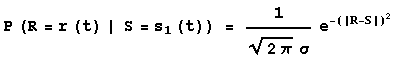Since the exponential function is monotonic, the decision metric can be further simplified as follows, assuming that the transmitted bit is a one when: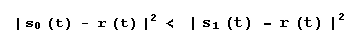This is conceptually intuitive, since this means that we choose a binary '1' when the received signal is 'closer' in distance to the waveform for a '1' than the waveform for a '0'. Also, the square terms can be expanded as follows: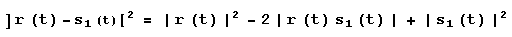Since the energy of the received signal is common to both sides of the decision metric, it can be dropped. By choosing the energy of the waveforms for each binary value to be identical, the energy of the signal terms cancel as well. Our decision metric is further simplified to choosing a binary '1' when: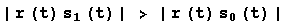This means that we should assume a binary one was transmitted when the correlation of the received signal with the waveform for a '1' is larger than the correlation with the other waveform. Again, this makes intuitive sense because the correlation operation is a measurement of similarity between two signals. The correlation operator can be expressed explicitly in its integral form as follows: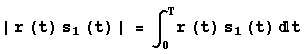Because we are dealing with digital, time discrete hardware, the integration is reduced to a sum. Finally, the metric used to determine whether what was received was a binary '0' or '1' is simply: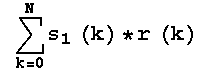If this value is greater that zero, we infer that a binary '1' was transmitted. Likewise, it the value is less than zero, we assume a binary '0' has been transmitted. This is the core of our receiver code.